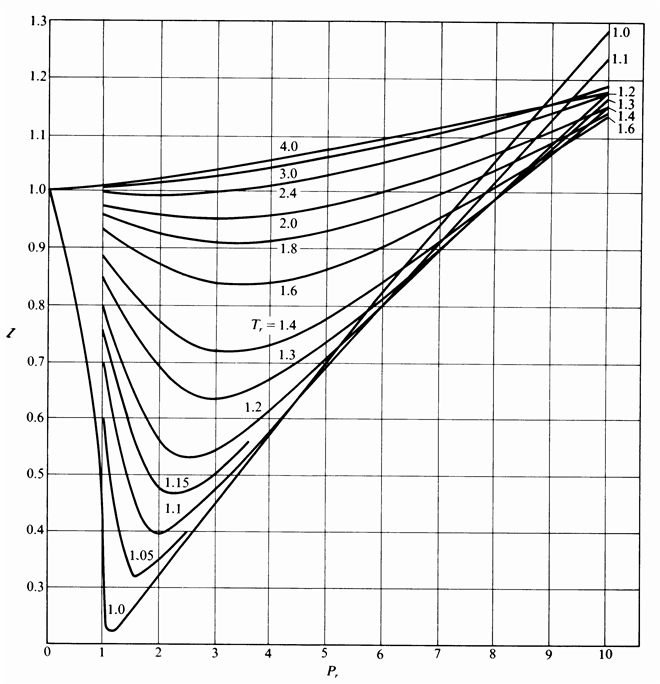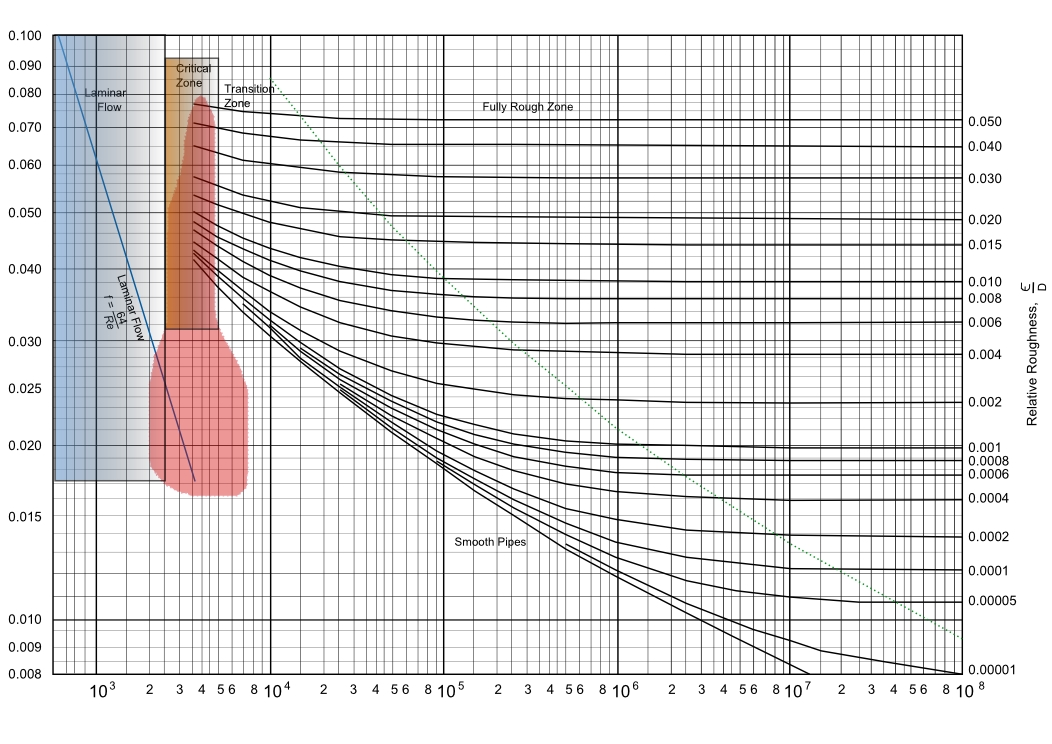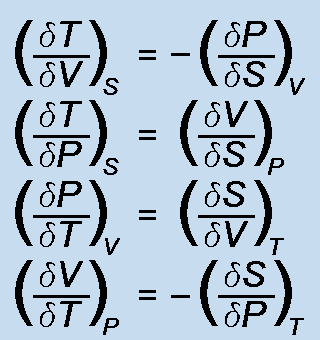### ChemE Soft StoreCaCO3 -> CaO + CO2
NaCl + NH3 + CO2 + H2O -> NaHCO3 + NH4Cl
2 NaHCO3 -> Na2 CO3 + H2O + CO2
CaO + H2 O -> Ca(OH)2
Ca(OH)2 + 2 NH4 Cl -> CaCl2 + 2 NH3 + 2 H2O

Vapor-Liq Equilibrium

Vapor-liquid equilibrium conditions are calculated for mixtures of up to six components. Compositions of either the liquid or vapor phase can be specified, from which the equilibrium phase conditions can be calculated. Flash calculations for incoming streams can also be solved.

Single Comp EOS

Nine different equations of state are used to calculate molecular volumes of both liquid and vapor phases at user specified temperatures and pressures. Also, equilibrium conditions can be calculated for actual two-phase systems. EOS's include Van der Waals, RK, SRK, PR, PRSV1 & 2, etc.

Multi Comp EOS

Six different cubic equations of state are used to calculate molecular volumes of mixtures for both liquid and vapor phases. EOS's include Van der Waals, RK, SRK, PR, PRSV1 and PRSV2.

### UNIFAC VLE

Vapor-Liquid equilibrium model for non-ideal mixtures using molecular functional groups to calculate activity co-efficients.